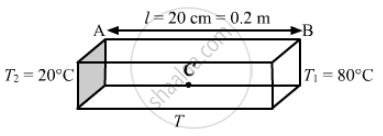Share

# The Left End of a Copper Rod (Length = 20 Cm, Area of Cross Section = 0.20 Cm2) is Maintained at 20°C and the Right End is Maintained at 80°C. - Physics

#### Question

The left end of a copper rod (length = 20 cm, area of cross section = 0.20 cm2) is maintained at 20°C and the right end is maintained at 80°C. Neglecting any loss of heat through radiation, find (a) the temperature at a point 11 cm from the left end and (b) the heat current through the rod. Thermal conductivity of copper = 385 W m−1°C−1.

#### SolutionArea of cross section, A = 0.2 cm2 = 0.2 × 10−4 m2
Thermal conductivity, k = 385 W m–1 °C–1

"Rate of flow of heat" = "temperature diffrences"/ "Thermal resistance"

(DeltaQ)/(Deltat) = (KA (T_1 - T_2))/ l

(DeltaQ)/(Deltat) = ((80 - 20 )/ 0.2) xx 385xx0.2xx10^-4

= 2310 × 10-3

= 2.31 J/sec

Let te temperature of point C be T, which is at a distance of 11 cm from the left end
Rate of flow of heat is given by

(DeltaQ)/(Deltat) = (KA DeltaT)/l

⇒ (DeltaT)/l= ((DeltaQ)/(Deltat))xx1/(KA)

(T-20)/(11xx10^-2) = 2.31/(383xx0.2xx10^-4)

⇒ T = 33 + 20

⇒ T = 53° c

Is there an error in this question or solution?

#### APPEARS IN

Solution The Left End of a Copper Rod (Length = 20 Cm, Area of Cross Section = 0.20 Cm2) is Maintained at 20°C and the Right End is Maintained at 80°C. Concept: Heat Transfer - Radiation.
S